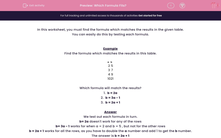# Which Formula Fits?

In this worksheet, students find the formula that matches the data in the given table.Key stage:  KS 2

Curriculum topic:   Maths and Numerical Reasoning

Curriculum subtopic:   Equations and Algebra

Difficulty level:#### Worksheet Overview

In this worksheet, you must find the formula which matches the results in the given table.

You can easily do this by testing each formula.

Example

Find the formula which matches the results in this table.

a b
2 5
3 7
4 9
10 21

Which formula will match the results?

1.  b = 2a

2.  b = 3a - 1

3.  b = 2a + 1

We test out each formula in turn.

b= 2a doesn't work for any of the rows

b= 3a - 1 works for when a = 2 and b = 5 , but not for the other rows

b = 2a + 1 works for all the rows, as you have to double the a number and add 1 to get the b number.

The answer is b = 2a + 1

### What is EdPlace?

We're your National Curriculum aligned online education content provider helping each child succeed in English, maths and science from year 1 to GCSE. With an EdPlace account you’ll be able to track and measure progress, helping each child achieve their best. We build confidence and attainment by personalising each child’s learning at a level that suits them.

Get started